# QUESTION 1 A sample of nitrogen gas is sealed into a 18.6 L container at 715.2...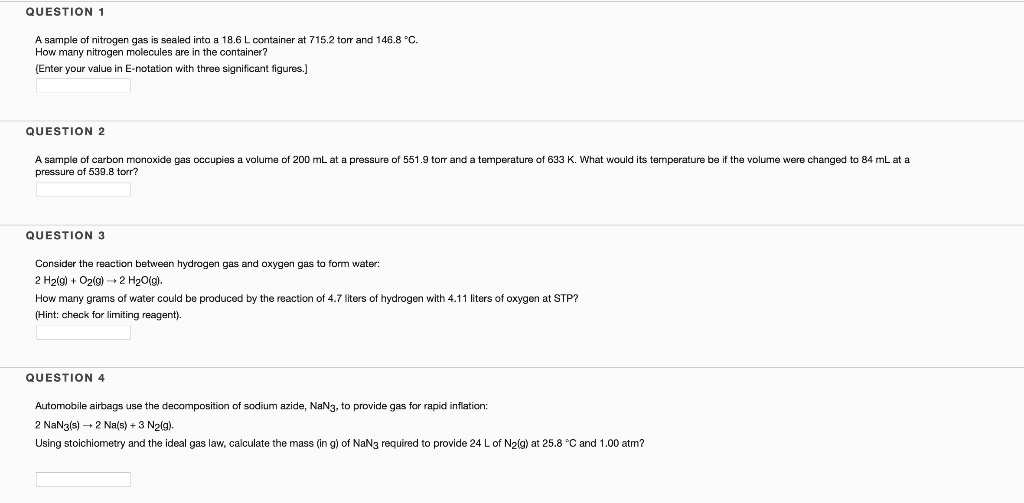QUESTION 1 A sample of nitrogen gas is sealed into a 18.6 L container at 715.2 torr and 146.8 °C. How many nitrogen molecules are in the container? (Enter your value in E-notation with three significant figures.] QUESTION 2 A sample of carbon monoxide gas occupies a volume of 200 mL at a pressure of 551.9 torr and a temperature of 633 K. What would its temperature be if the volume were changed to 84 mL at a pressure of 539.8 torr? QUESTION 3 Consider the reaction between hydrogen gas and oxygen gas to form water: 2 H2(g) + O2(g) + 2 H2O(g). How many grams of water could be produced by the reaction of 4.7 liters of hydrogen with 4.11 liters of oxygen at STP? (Hint: check for limiting reagent). QUESTION 4 Automobile airbags use the decomposition of sodium azide, NaN3, to provide gas for rapid inflation: 2 NaN3(s) + 2 Na(s) + 3 N2(g). Using stoichiometry and the ideal gas law, calculate the mass (in g) of NaNg required to provide 24 L of N2(g) at 25.8 °C and 1.00 atm?

1)

Given:

P = 715.2 torr

= (715.2/760) atm

= 0.9411 atm

V = 18.6 L

T = 146.8 oC

= (146.8+273) K

= 419.8 K

find number of moles using:

P * V = n*R*T

0.9411 atm * 18.6 L = n * 0.08206 atm.L/mol.K * 419.8 K

n = 0.5081 mol

use:

number of molecules = number of mol * Avogadro’s number

number of molecules = 0.5081 * 6.022*10^23 molecules

number of molecules = 3.06*10^23 molecules

Only 1 question at a time please

#### Earn Coin

Coins can be redeemed for fabulous gifts.

Similar Homework Help Questions
• ### question 1-4 please :) A sample of nitrogen gas is sealed into a 14.7L container at...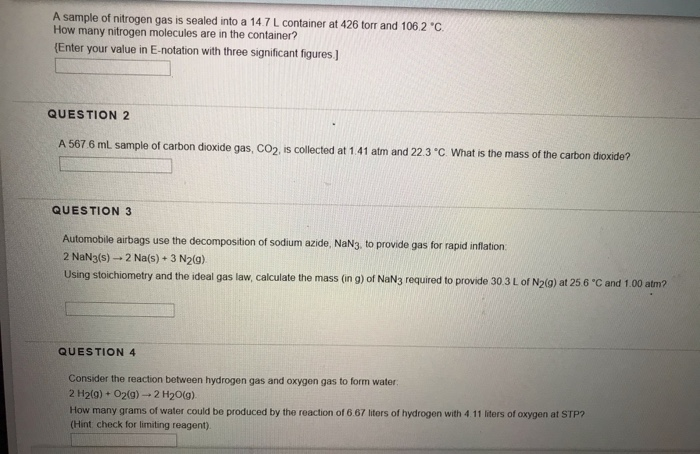question 1-4 please :) A sample of nitrogen gas is sealed into a 14.7L container at 426 torr and 106 2 °C How many nitrogen molecules are in the container? {Enter your value in E-notation with three significant figures.] QUESTION 2 A 567.6 ml sample of carbon dioxide gas, CO2, is collected at 1.41 atm and 22.3°C. What is the mass of the carbon dioxide? QUESTION 3 Automobile airbags use the decomposition of sodium azide, NaN3, to provide gas for...

• ### QUESTION 2 A sample of nitrogen gas is sealed into a 18.6 L container at 715.2 torr and 146.8 °C. How many nitrogen...QUESTION 2 A sample of nitrogen gas is sealed into a 18.6 L container at 715.2 torr and 146.8 °C. How many nitrogen molecules are in the container? (Enter your value in E-notation with three significant figures.]

• ### QUESTION 1 What will be the pressure of 52.5 grams of oxygen gas in a glass...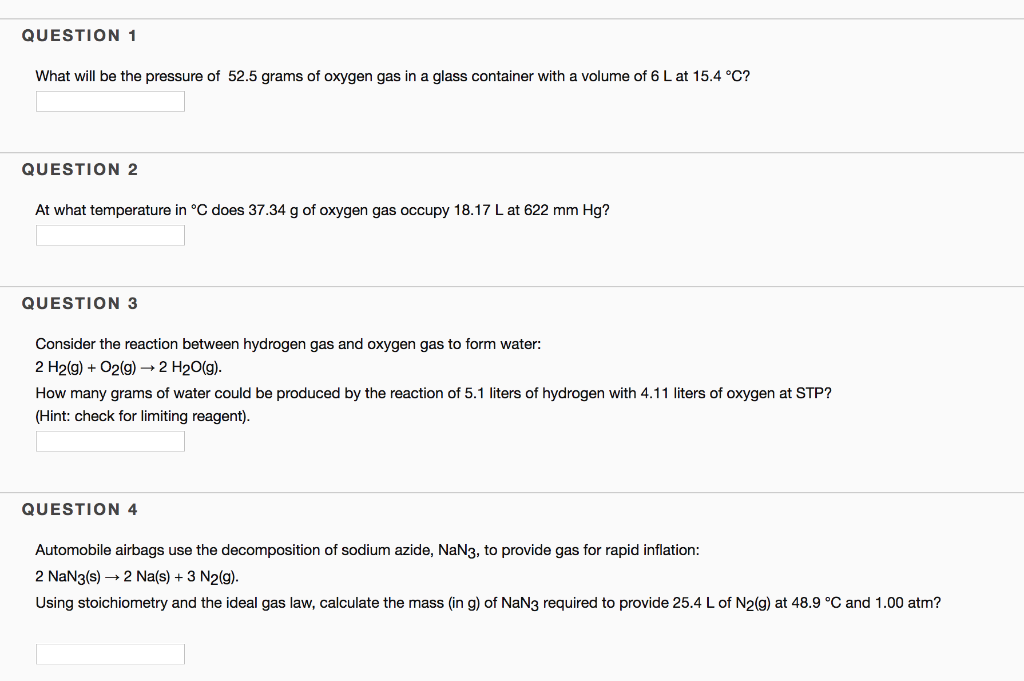QUESTION 1 What will be the pressure of 52.5 grams of oxygen gas in a glass container with a volume of 6 L at 15.4 °C? QUESTION 2 At what temperature in °C does 37.34 g of oxygen gas occupy 18.17 L at 622 mm Hg? QUESTION 3 Consider the reaction between hydrogen gas and oxygen gas to form water: 2 H2(g)O2(g)-»2 H20(g) How many grams of water could be produced by the reaction of 5.1 liters of hydrogen with...

• ### QUESTION 1 0.5 points Save Answer A 567.6 mL sample of carbon dioxide gas, CO2, is...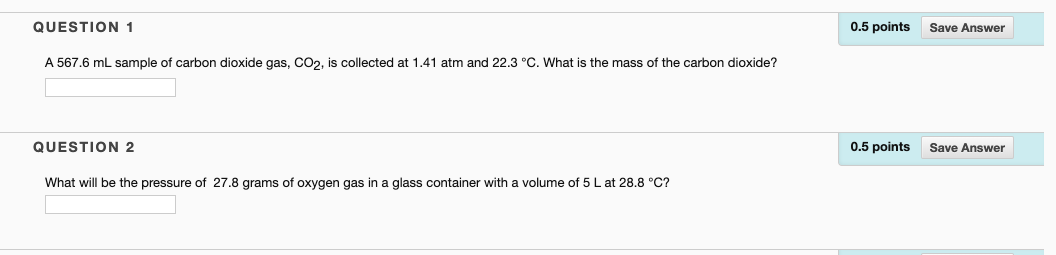QUESTION 1 0.5 points Save Answer A 567.6 mL sample of carbon dioxide gas, CO2, is collected at 1.41 atm and 22.3 °C. What is the mass of the carbon dioxide? QUESTION 2 0.5 points Save Answer What will be the pressure of 27.8 grams of oxygen gas in a glass container with a volume of 5 L at 28.8 °C? QUESTION 3 0.5 points Save Answer Consider the reaction between hydrogen gas and oxygen gas to form water: 2...

• ### Please help with these two questions 1.) Consider the reaction between hydrogen gas and oxygen gas...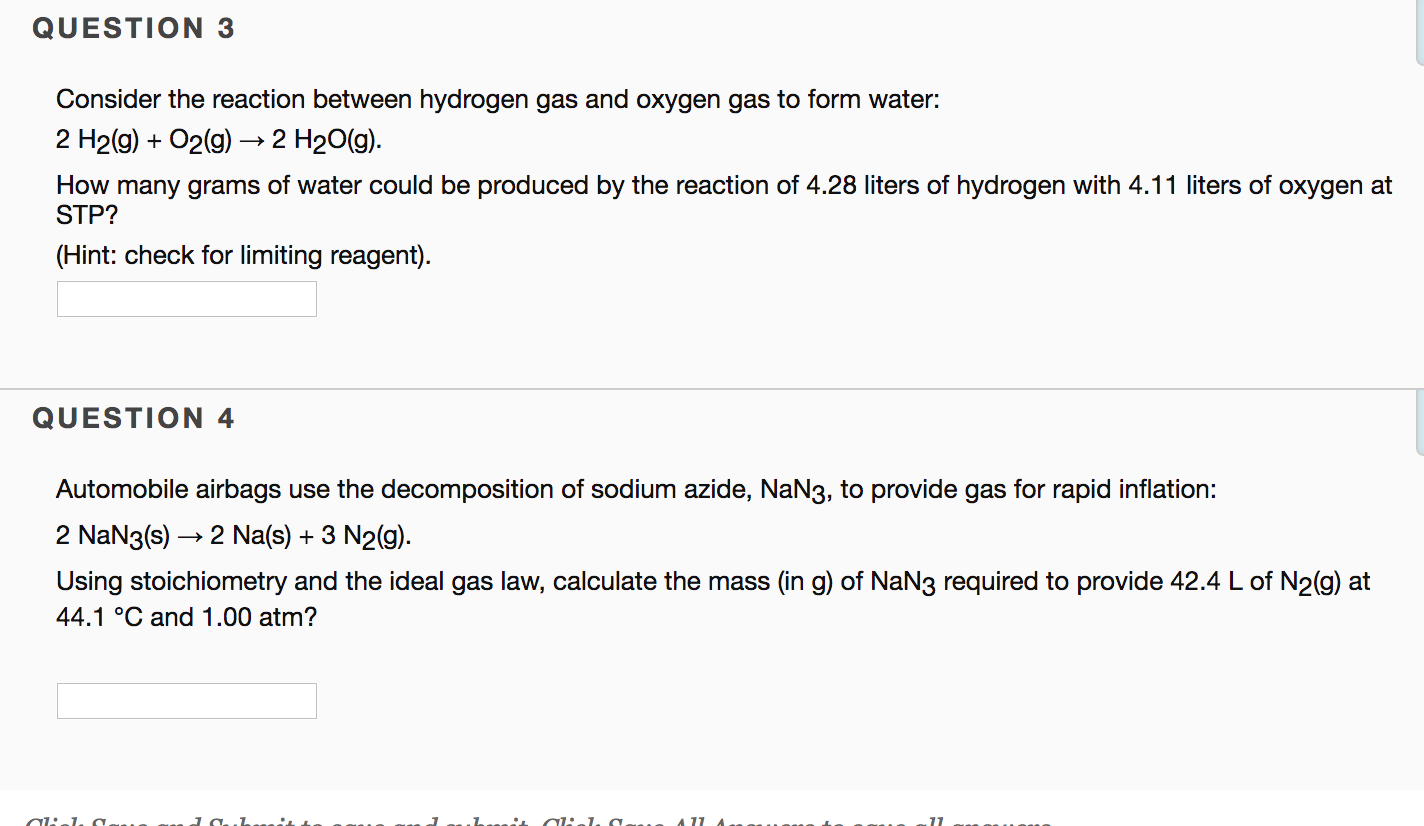Please help with these two questions 1.) Consider the reaction between hydrogen gas and oxygen gas to form water: 2 H2(g) + O2(g) → 2 H2O(g). How many grams of water could be produced by the reaction of 4.28 liters of hydrogen with 4.11 liters of oxygen at STP? 2.) Automobile airbags use the decomposition of sodium azide, NaN3, to provide gas for rapid inflation: 2 NaN3(s) → 2 Na(s) + 3 N2(g). Using stoichiometry and the ideal gas law,...

• ### QUESTION 3 Automobile airbags use the decomposition of sodium azide, NaN3, to provide gas for rapid...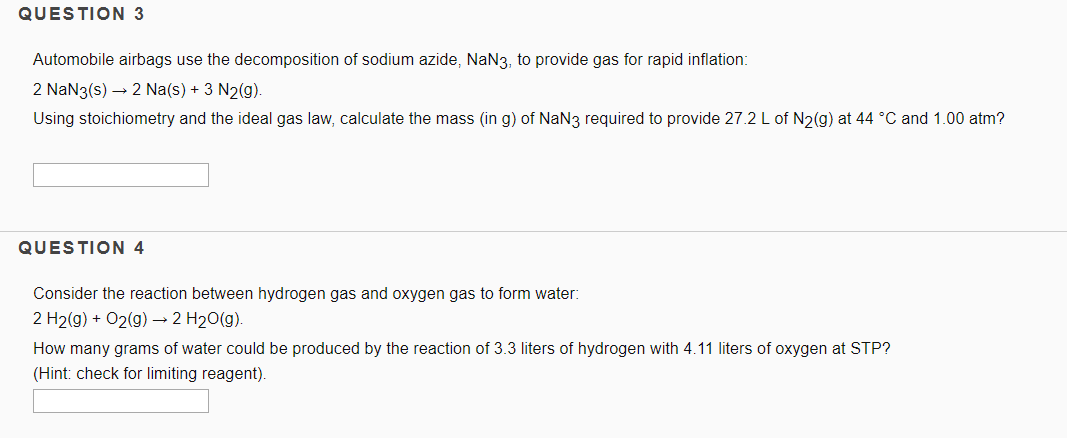QUESTION 3 Automobile airbags use the decomposition of sodium azide, NaN3, to provide gas for rapid inflation: 2 NaN3(s) → 2 Na(s) + 3 N2(g). Using stoichiometry and the ideal gas law, calculate the mass (in g) of NaN3 required to provide 27.2 L of N2(g) at 44 °C and 1.00 atm? QUESTION 4 Consider the reaction between hydrogen gas and oxygen gas to form water: 2 H2(g) + O2(g) + 2 H20(9). How many grams of water could be...

• ### Automobile airbags use the decomposition of sodium azide, NaN3, to provide gas for rapid inflation: 2...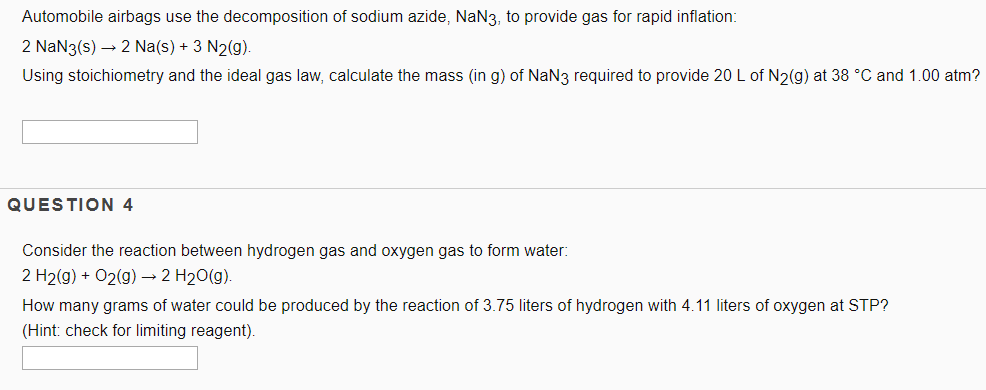Automobile airbags use the decomposition of sodium azide, NaN3, to provide gas for rapid inflation: 2 NaN3(s) – 2 Na(s) + 3 N2(9). Using stoichiometry and the ideal gas law, calculate the mass (in g) of NaN3 required to provide 20 L of N2(g) at 38 °C and 1.00 atm? QUESTION 4 Consider the reaction between hydrogen gas and oxygen gas to form water: 2 H2(g) + O2(g) → 2 H2O(g). How many grams of water could be produced by...

• ### 3&4 QUESTION 3 Automobile airbags use the decomposition of sodium azide, NaN3, to provide gas for...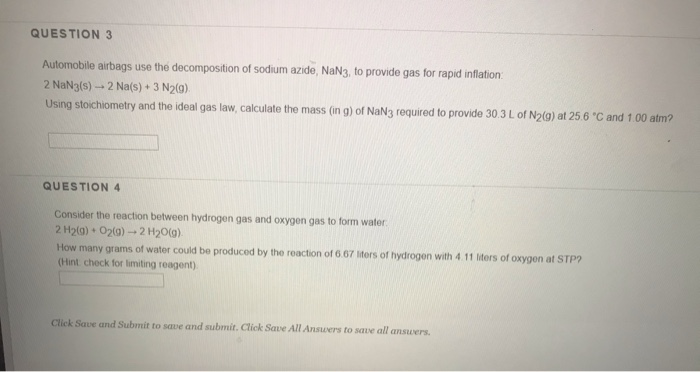3&4 QUESTION 3 Automobile airbags use the decomposition of sodium azide, NaN3, to provide gas for rapid inflation: 2 NaN3(s) 2 Na(s) + 3 N2(g) Using stoichiometry and the ideal gas law, calculate the mass (in g) of NaN3 required to provide 30.3 L of N2(g) at 25.6 C and 1 00 atm? QUESTION 4 Consider the reaction between hydrogen gas and oxygen gas to form water 2 H2(g) O2(a)-2 H20(g) How many grams of water could be produced by...

• ### answer both questions pls!! QUESTION 3 Automobile airbags use the decomposition of sodium azide, NaN3, to...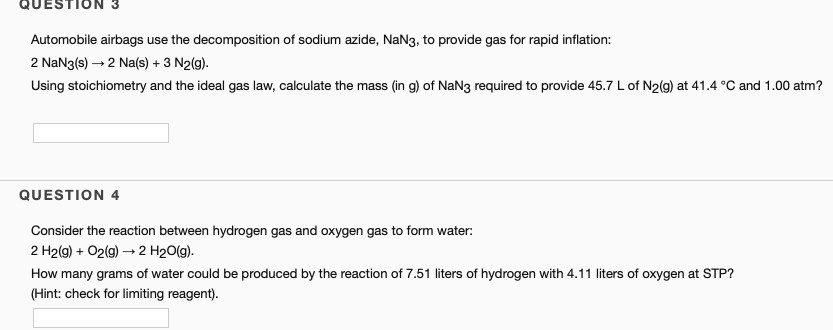answer both questions pls!! QUESTION 3 Automobile airbags use the decomposition of sodium azide, NaN3, to provide gas for rapid inflation: 2 NaN3(s) + 2 Na(s) + 3 N2(g). Using stoichiometry and the ideal gas law, calculate the mass (in g) of NaN3 required to provide 45.7 L of N2(g) at 41.4 °C and 1.00 atm? QUESTION 4 Consider the reaction between hydrogen gas and oxygen gas to form water: 2 H2(g) + O2(g) + 2 H2O(g). How many grams...

• ### The air bags in automobiles were once inflated by nitrogen gas generated by the rapid decomposition...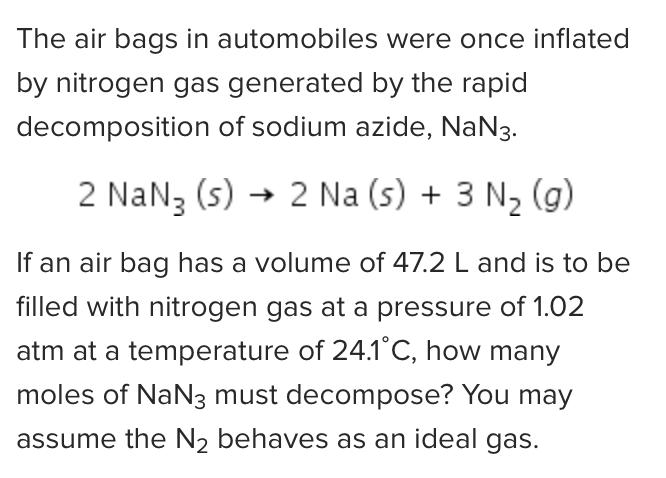The air bags in automobiles were once inflated by nitrogen gas generated by the rapid decomposition of sodium azide, NaN3. 2 NaNz (s) + 2 Na (s) + 3 N2(g) If an air bag has a volume of 47.2 L and is to be filled with nitrogen gas at a pressure of 1.02 atm at a temperature of 24.1°C, how many moles of NaN3 must decompose? You may assume the N2 behaves as an ideal gas.